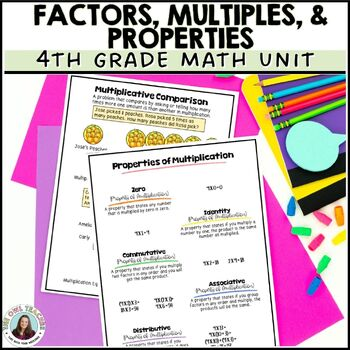# Multiplication & Division Basics Guided Math Workshop Grade 4

Rated 4.5 out of 5, based on 2 reviews
2 Ratings;
4th
Subjects
Resource Type
Standards
Formats Included
• PDF
•Google Apps™
Pages
201+
\$12.00
\$12.00
Report this resource to TPTThe Teacher-Author indicated this resource includes assets from Google Workspace (e.g. docs, slides, etc.).

### Description

It's important that students know have fact fluency with accuracy. This multiplication and division basics resource will help your students do just that while meeting other important standards. It's all about building those important math foundations.

Save 30% off now and buy the year-long bundle!

This guided math workshop unit is complete with pre-tests, vocabulary cards, 15 days of lesson plans that are very thorough and detailed, guided math activities, interventions and extension suggestions, math centers (such as math games), post-tests for math assessment, and much more!

You can also get a free sample of one of my math workshop units for third grade here.

Check out the preview for details!

This Unit Covers:

• Factors
• Multiples
• Ways to remember the difference between factors and multiples
• Divisibility rules
• prime and composite numbers
• strategies for multiplication and division (equal grouping, arrays, repeated addition, repeated subtraction, skip-counting)
• The terms for multiplication and division parts (divisor, dividend, quotient & factor, product)
• multiplicative comparison problems
• addition comparison vs multiplicative comparison
• Properties of multiplication (zero property, identity property, commutative property, associative property & distributive property)
• Focus on distributive property
• Patterns in multiplication (multiples of 10)
• Patterns and finishing sequences
• Input-output tables / functioning machines
• Determining the rule in patterns

With This Unit You'll Get:

• Pre-test and post-test
• Vocabulary cards for math word wall instruction
• Detailed lesson plans
• Anchor charts
• Differentiated math activities
• Interactive notebook pieces (INB)
• Math centers or math stations materials
• Math games
• Math worksheets for extra practice & review
• Remediation & enrichment
• Mini-assessments
• Digital & print versions
• Color & printer-friendly versions

Other Related Products You May Enjoy:

Multi-digit Multiplication for Math Workshop

Multi-digit Division for Math Workshop

Addition & Subtraction Math Workshop (4th Gr)

**IMPORTANT NOTICE**

This resource is not editable. I cannot allow the resource to be editable due to the Terms of Use on the clip art used. Thank you for understanding.

Printing Notes:

This resource is printer-friendly and includes a black and white version. I would suggest printing "as image" to avoid any potential issues. Please also make sure your adobe reader (or the like program) is up-to-date.

Mistakes Happen!☜

If you see ANYTHING that needs modifying or if you have any questions, please contact me via the Q&A. I take my product creation and your satisfaction very seriously! Thanks!

Earn FREE Resources!

It's very important to me that you provide feedback so that I may improve and create products you will use and love! Please consider leaving detailed feedback. Additionally, each time you provide feedback, you earn TpT credits. These can be taken off purchases so you can get items free!

❤️Love What You See? ❤️

Join my email list here to get FREEBIES and so much more!

Follow my store here to get discounted goodies each time they're posted!

Keywords: Multiplication games, Multiplication facts practice, multiplication practice, math games, multiplication review, Multiplication Worksheets, Multiplication Centers, Basic Facts, Basic Multiplication, factors, factors and multiples, divisibility rules, divisibility rules activities, multiplicative comparison, properties of addition and multiplication, properties of multiplication, distributive property, multiplication and division, prime and composite numbers, number patterns, input output tables, math workshop, guided math, lesson plans

Total Pages
201+
Included with rubric
Teaching Duration
3 Weeks
Report this resource to TPT
Reported resources will be reviewed by our team. Report this resource to let us know if this resource violates TPT’s content guidelines.

### Standards

to see state-specific standards (only available in the US).
Multiply a whole number of up to four digits by a one-digit whole number, and multiply two two-digit numbers, using strategies based on place value and the properties of operations. Illustrate and explain the calculation by using equations, rectangular arrays, and/or area models.
Find whole-number quotients and remainders with up to four-digit dividends and one-digit divisors, using strategies based on place value, the properties of operations, and/or the relationship between multiplication and division. Illustrate and explain the calculation by using equations, rectangular arrays, and/or area models.
Interpret a multiplication equation as a comparison, e.g., interpret 35 = 5 × 7 as a statement that 35 is 5 times as many as 7 and 7 times as many as 5. Represent verbal statements of multiplicative comparisons as multiplication equations.
Multiply or divide to solve word problems involving multiplicative comparison, e.g., by using drawings and equations with a symbol for the unknown number to represent the problem, distinguishing multiplicative comparison from additive comparison.
Find all factor pairs for a whole number in the range 1-100. Recognize that a whole number is a multiple of each of its factors. Determine whether a given whole number in the range 1-100 is a multiple of a given one-digit number. Determine whether a given whole number in the range 1-100 is prime or composite.